/

### Function Description

Returns the weighted Spearman correlation between two series.

The weighted Spearman rank correlation for two series, 1 and 2, given weights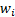(for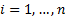), is calculated as: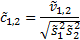where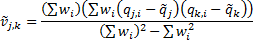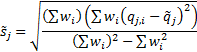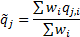and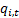are the ranks of the values in the series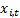, i.e.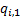is the largest value in the series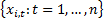See Weighted Moments and Cumulants for further details and explanation of terms in this formula. If the weights are equal then returns the same as MnSpearmanRankCorrelation.

Contents | Prev | Next